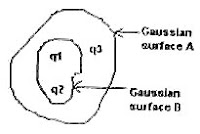## Wednesday, April 25, 2007

### Three Karnataka CET 2005 Questions on ElectrostaticsThe following three questions which appeared in Karnataka CET 2005 are aimed at testing your understanding of basic principles in electrostatics.

(1) The electric flux for Gaussian surface A that encloses the charged particles in free space is ( given that q1 = –14 nC, q2 = 78.85 nC, q3 = – 56 nC)

(a) 103 Nm2C–1 (b) 103 CN–1m–2

(c) 6.32×103 Nm2C–1 (d) 6.32×103 CN–1m–2

The Gaussian surface B is just for distracting you. Remember, Gaussian surface is an imagined surface and it has no action on the charge configuration. When you consider the Gaussian surface A, the net charge enclosed by the surface is –14 nC + 78.85 nC + (– 56 nC) = 8.85 nC.

The electric flux over the closed surface A, according to Gauss theorem, is q/ε0 where q is the net charge enclosed by the surface.

Therefore, electric flux = 8.85×10–9/8.85×10–12 = 103 Nm2C–1 [Option (a)].

[You should note that the electric flux is the product of electric field and area so that its unit is (N/C)×m2 = Nm2C–1].

(2) The work done in carrying a charge ‘q’ once round a circle of radius ‘r’ with a charge ‘Q’ at the centre is

(a) qQ/4πε0r (b) qQ/4πε02r2 (c) qQ/4πε0r2 (d) none of these

The work done is zero since there is no potential difference between the initial and final positions of the charge q. The correct option therefore is (d). Note that this is the case for any closed path of any shape.

(3) An air filled parallel plate condenser has a capacity of 2 pF. The separation of the plates is doubled and the inter space between the plates is filled with wax. If the capacity is increased to 6 pF, the dielectric constant of wax is

(a) 2 (b) 3 (c) 4 (d) 6

The capacitance of air cored capacitor is ε0A/d where A is the area of the plates and ‘d’ is the separation between the plates. When the separation is doubled, the capacitance is halved and becomes 1 pF.

The capacitance when the inter space is filled with a dielectric of dielectric constant ‘K’, the capacitance is Kε0A/d so that it is increased to K times the value with air as the dielectric. Since the increment is from 1 to 6, K = 6.